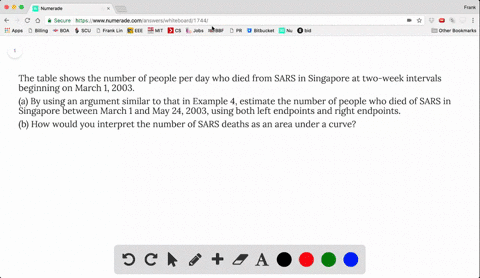### The table shows the number of people per day who …

02:01University of California, Berkeley
Problem 19

In someone affected with measles, the virus level $N$ (measured in number of infected cells per mL of blood plasma) reaches a peak density at about $t = 12$ days (when a rash appears) and then decreases fairly rapidly as a result of immune response. The area under the graph of $N(t)$ from
$t = 0$ to $t = 12$ (as shown in the figure) is equal to the total amount of infection needed to develop symptoms (measured in density of infected cells x time). The function $N$ has been modeled by the function $$f(t) = -t(t - 21)(t + 1)$$

Use this model with six subintervals and their midpoints to estimate the total amount of infection needed to develop symptoms of measles.

7840

## Discussion

You must be signed in to discuss.

## Video Transcript

Okay. First thing we could do is calculate changing Axe 12 months years, right by sex is 12 over six, which is simply too two times off of one. That's 40 plus half of 3 to 16 plus of a five for 80 post up of 77 84 puts up of 9 1080 was half of 11 1320 gives us 78 40 is our solution.